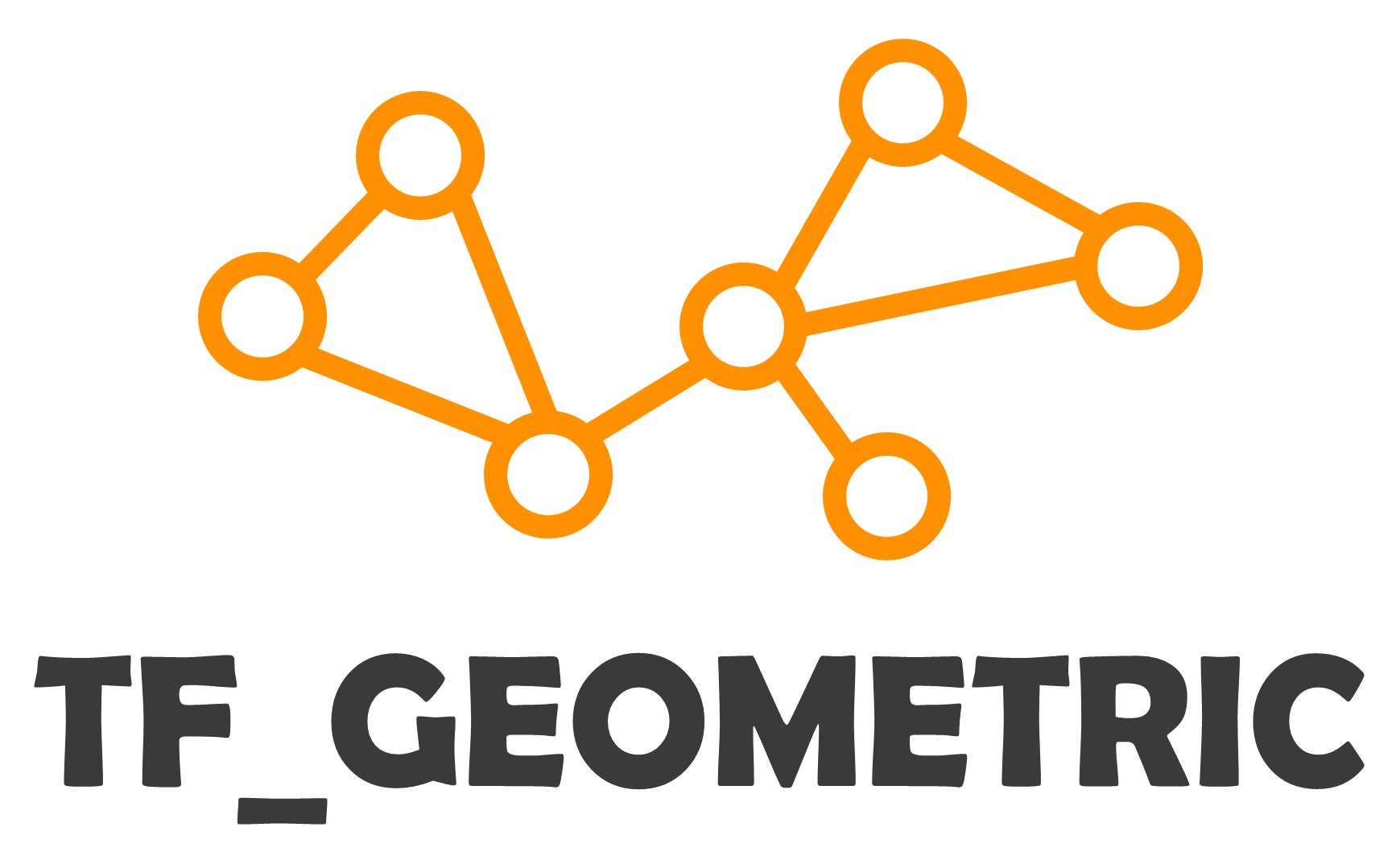# tf_geometric Documentation¶

(中文版)

Efficient and Friendly Graph Neural Network Library for TensorFlow 1.x and 2.x.

Inspired by rusty1s/pytorch_geometric, we build a GNN library for TensorFlow. tf_geometric provides both OOP and Functional API, with which you can make some cool things.## Efficient and Friendly API¶

We use Message Passing mechanism to implement graph neural networks, which is way efficient than the dense matrix based implementations and more friendly than the sparse matrix based ones. In addition, we provide easy and elegant APIs for complex GNN operations. The following example constructs a graph and applies a Multi-head Graph Attention Network (GAT) on it:

```# coding=utf-8
import numpy as np
import tf_geometric as tfg
import tensorflow as tf

graph = tfg.Graph(
x=np.random.randn(5, 20),  # 5 nodes, 20 features,
edge_index=[[0, 0, 1, 3],
[1, 2, 2, 1]]  # 4 undirected edges
)

print("Graph Desc: \n", graph)

graph.convert_edge_to_directed()  # pre-process edges
print("Processed Graph Desc: \n", graph)
print("Processed Edge Index:\n", graph.edge_index)

# Multi-head Graph Attention Network (GAT)
output = gat_layer([graph.x, graph.edge_index])
print("Output of GAT: \n", output)
```

Output:

```Graph Desc:
Graph Shape: x => (5, 20)  edge_index => (2, 4)    y => None

Processed Graph Desc:
Graph Shape: x => (5, 20)  edge_index => (2, 8)    y => None

Processed Edge Index:
[[0 0 1 1 1 2 2 3]
[1 2 0 2 3 0 1 1]]

Output of GAT:
tf.Tensor(
[[0.22443159 0.         0.58263206 0.32468423]
[0.29810357 0.         0.19403605 0.35630274]
[0.18071976 0.         0.58263206 0.32468423]
[0.36123228 0.         0.88897204 0.450244  ]
[0.         0.         0.8013462  0.        ]], shape=(5, 4), dtype=float32)
```

## Tutorial¶

### Getting Started with Demo¶

We recommend you to get started with some demo.

## Cite¶

If you use tf_geometric in a scientific publication, we would appreciate citations to the following paper:

```@inproceedings{DBLP:conf/mm/HuQFWZZX21,
author    = {Jun Hu and
Shengsheng Qian and
Quan Fang and
Youze Wang and
Quan Zhao and
Huaiwen Zhang and
Changsheng Xu},
editor    = {Heng Tao Shen and
Yueting Zhuang and
John R. Smith and
Yang Yang and
Pablo Cesar and
Florian Metze and
Balakrishnan Prabhakaran},
title     = {Efficient Graph Deep Learning in TensorFlow with tf{\_}geometric},
booktitle = {{MM} '21: {ACM} Multimedia Conference, Virtual Event, China, October
20 - 24, 2021},
pages     = {3775--3778},
publisher = {{ACM}},
year      = {2021},
url       = {https://doi.org/10.1145/3474085.3478322},
doi       = {10.1145/3474085.3478322},
timestamp = {Wed, 20 Oct 2021 12:40:01 +0200},
biburl    = {https://dblp.org/rec/conf/mm/HuQFWZZX21.bib},
bibsource = {dblp computer science bibliography, https://dblp.org}
}
```